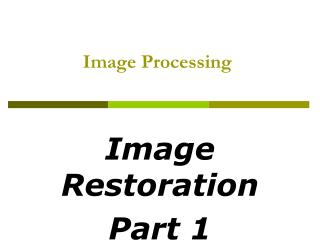DownloadDownload PresentationImage Processing

# Image Processing

Download Presentation## Image Processing

- - - - - - - - - - - - - - - - - - - - - - - - - - - E N D - - - - - - - - - - - - - - - - - - - - - - - - - - -
##### Presentation Transcript

1. Image Processing Image Restoration Part 1

2. What is Image Restoration? • Image restoration attempts to restore images that have been degraded by using a prior knowledge of the degradation phenomenon. • Identify the degradation process and attempt to reverse it • Similar to image enhancement, but more objective

3. Noise Model • We can consider a noisy image to be modelled as follows: • where f(x, y) is the original image pixel, η(x, y) is the noise term and g(x, y) is the resulting noisy pixel • If we can estimate the model the noise in an image is based on this will help us to figure out how to restore the image

4. Degradation/restoration process model • Degradation model • A degradation function and additive noise that operate on an input image f(x, y) to produce a degraded image g(x, y):

5. Degradation/restoration process model • Restoration model • Given g(x, y) and some knowledge about the degradation function H and the noise η, obtain an estimate fˆ(x, y) of the original image • If H is a linear spatially invariant process: • In spatial domain: • h(x, y): spatial representation of the degradation function • In an equivalent frequency domain

6. Sources of noise • Arise during image acquisition (digitization) and/or transmission • Environmental conditions: light, temperature, humidity, atmospheric disturbance... • Quality of sensing elements and transmission media • Human interference • Assumptions of noise • Independent of spatial coordinates • Uncorrelated with respect to the image

7. Noise Model • Spatial noise • Considered as random variables, characterized by a probability density function (PDF) • Noise models • Simulate the behavior and effect of noise • There are many different models for the image noise term η(x, y) • Gaussian • Most common model • Rayleigh • Erlang • Exponential • Uniform • Impulse • Salt and pepper noise

8. Noise Model • Gaussian (normal) noise, PDF: * z: gray level, μ: mean value, σ: standard deviation,σ2: variance

9. Noise Model

10. Noise Model

11. Noise Model

12. Histogram to go here Noise Example • The test pattern to the right is ideal for demonstrating the addition of noise • Consists of constant areas that span the gray scale from black to near white. Image Histogram

13. Noise Example (cont…)

14. Noise Example (cont…)

15. Periodic Noise • Typically arises from electrical or electromechanical interference during image acquisition. • FT of a pure sinusoid is a pair of conjugate impulses located at the conjugate frequencies of sine wave. • More in section 5.4

16. Periodic Noise

17. Estimation of Noise Parameters • The parameters of noise PDFs may be known partially from sensor specifications • But its necessary to estimate them form images. • Capture a set of images of flat environment. • In the case of optical sensors, this is simple as imaging a solid board that is illuminated uniformly. • The resulting images are good indicators of system noise.

18. Estimation of Noise Parameters • Use data from image strip to calculate the mean and variance of the gray levels. • If the strip is denoted by S, then

19. Restoration in the Presence of Noise Only-Spatial Filtering • When the only degradation present in an image is noise, • and • The noise terms are unknown, so subtracting them from g(x,y) or G(u,v) is not realistic option. • In periodic noise, it is possible to estimate N(u,v) from the spectrum of G(u,v). • So subtraction can be done to obtain an estimate of the original image • This is an exception rather than a rule • Spatial filtering is the method of choice in situations when only additive noise is present.

20. ** Impulsive (Salt & Pepper) Noise • Definition • Each pixel in an image has a probability pa or pb of being contaminated by a white dot (salt) or a black dot (pepper) X: noise-free image, Y: noisy image with probability pa noisy pixels with probability pb clean pixels with probability 1 - pa - pb add salt & pepper noise